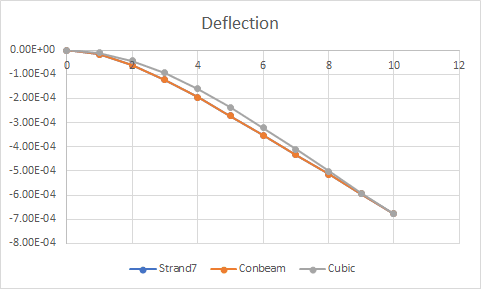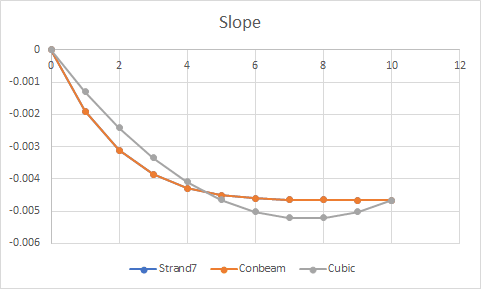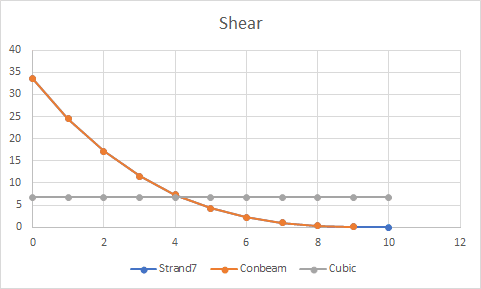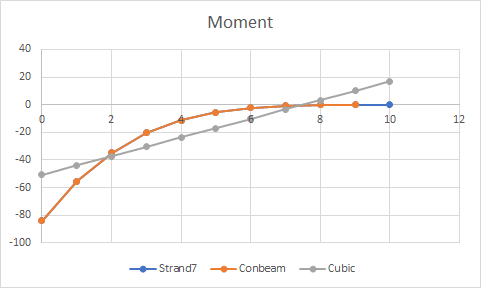×
INTELLIGENT WORK FORUMS
FOR ENGINEERING PROFESSIONALS

Are you an
Engineering professional?
Join Eng-Tips Forums!
• Talk With Other Members
• Be Notified Of Responses
• Keyword Search
Favorite Forums
• Automated Signatures
• Best Of All, It's Free!

*Eng-Tips's functionality depends on members receiving e-mail. By joining you are opting in to receive e-mail.

#### Posting Guidelines

Promoting, selling, recruiting, coursework and thesis posting is forbidden.

# 1-FEM-Element vs Analytical Solution of a Cantilever Euler-Bernoulli Beam - Theoretical Background

## 1-FEM-Element vs Analytical Solution of a Cantilever Euler-Bernoulli Beam - Theoretical Background

(OP)
Hi,

I have been reading simple example problems of FEM vs analytical solutions, and I run into a very simple (and maybe stupid) doubt which answer I couldn't find over days after searching in FEM textbooks!

Let's assume we have a classical cantilever Euler-Bernoulli beam, clamped at the root and free at the tip. We know the classical governing equation for this problem according to Strength of Materials is a 4th order differential equation: EI d^4(v(x))/dx^4 - q(x) = 0

If there is no distributed load, the solution of the displacements in the interior of the beam will be a 3th order polynomial. Furthermore, if we want to solve it adopting a classical 1-FEM-element composed of 2 nodes, in which Hermite Polynomials are formulated and a cubic polynomial is defined for the displacements function v(x) (4 parameters to get constrains of vertical displacements and rotations), the FEM will lead to the exact solution. Both v(x)_FEM v(x)_exact will match.

However, if the distributed load is constant, linear, quadratic, and so on... and if we want to solve it adopting a classical 1-FEM-element with 2 nodes, the displacements at the interior of the element v(x)_FEM will no longer match the analytical solution v(x)_exact.

For instance, assuming a distributed load q(x) of quadratic form, the analytical solution of v(x)_exact will be a 6th order polynomial, against the same cubic Hermite polynomials for v(x)_FEM. Obviously, there will be an error on the solution, and even worse for forces, moments, stresses, strains since the errors will magnify when differentiating from v(x). However... the displacements computed at the tip node will still match between 1-FEM-element and the analytical solution!

Why is this happening? What am I missing? Is it related to the weak form formulation of the Finite Element Method and the essential/natural BCs?

Thanks.

Francesco

### RE: 1-FEM-Element vs Analytical Solution of a Cantilever Euler-Bernoulli Beam - Theoretical Background

One way to think of it is that the an element doesn't really understand distributed loads, they are applied at the nodes. Back in the day we actually had to write macros to turn distributed loads into nodal loads.

Cheers

Greg Locock

New here? Try reading these, they might help FAQ731-376: Eng-Tips.com Forum Policies http://eng-tips.com/market.cfm?

### RE: 1-FEM-Element vs Analytical Solution of a Cantilever Euler-Bernoulli Beam - Theoretical Background

Due to the way distributed loads are treated in FEM (converted to nodal forces) a single beam element with 2 nodes is simply useless for cases involving this type of loads. It just can’t properly capture their effects.

### RE: 1-FEM-Element vs Analytical Solution of a Cantilever Euler-Bernoulli Beam - Theoretical Background

(OP)
Hi,

Thanks to both for the prompt response!

@FEA_way, if a 1-beam-element with 2 nodes is modeled, and the distributed vertical load is quadratic, the solution for displacement matches with the exact theory at the tip!

My question was focused on the mathematical reason (provided that both v(x)_FEM and v(x)_exact are polynomials of 3rd and 6th order respectively).

Francesco.

### RE: 1-FEM-Element vs Analytical Solution of a Cantilever Euler-Bernoulli Beam - Theoretical Background

Yes, but all the remaining output variables (stress, strain, reactions) are incorrect, as are the displacements in other locations than the tip of the beam, right ? To me, this rules the aforementioned element out of use for distributed loads (unless more of these elements are utilized to mesh the beam, of course).

### RE: 1-FEM-Element vs Analytical Solution of a Cantilever Euler-Bernoulli Beam - Theoretical Background

To expand on Greg's response, the equations that are solved in a frame analysis have only the following information:
- The stiffness of the nodes (i.e. the ends of all the beams) to translation and rotation.
- The forces and moments applied to each node.

The analysis neither knows nor cares what happens in between the nodes, it is concerned only with the interaction of nodes, but if the actions at the nodes have been calculated correctly, then the displacement and rotation of every node will be exactly as defined by whatever beam bending theory was used to define the stiffness of the nodes.

An analogy I find useful is fitting a cubic spline to a higher order polynomial. To do this, points are selected along the curve, including the two ends, and the curvature at the two ends is specified. The fitting process will then produce a series of cubic equations that pass through the specified points exactly. The two lines won't match anywhere else, but the spline provides a useful approximation.

Regarding the forces, stresses and strains between the nodes, it is true that if the cubic equation is used these will not match the actual beam, but there is no reason to do that. Knowing the forces and moments at each end of the beam, and the distribution of loads along the beam, it is a simple matter to calculate the actual actions along the beam, and these will match the "exact" actions of the applied theory.

#### Quote (GregLocock)

Back in the day we actually had to write macros to turn distributed loads into nodal loads.

And for those of us whose hobby is writing FEA programs in Excel, we still do :)

https://newtonexcelbach.com/2009/07/05/continuous-...

Doug Jenkins
Interactive Design Services
http://newtonexcelbach.wordpress.com/

### RE: 1-FEM-Element vs Analytical Solution of a Cantilever Euler-Bernoulli Beam - Theoretical Background

you are running "single element patch" tests, an excellent thing to do when picking up FEA. With very simple loads and constraints you are testing the element coding to see how well it corresponds to "reality". Different source codes and different BEAM elements within a code will give different results, some better than others at representing "reality".

In you simple cantilever test you should see the reactions balance the applied load (no surprise there).
The displacement of tip tells you how well the element stiffness is modelled. As you've seen it differs for the load applied (correct for a distributed load, less correct for a point load.
The internal stresses are a different issue, I would suspect that the raw results (at the integration points may not be what you expect, but maybe the extrapolation to the ends is ?

### RE: 1-FEM-Element vs Analytical Solution of a Cantilever Euler-Bernoulli Beam - Theoretical Background

I think everyone is misunderstanding the question. He clearly knows and understands why the FEM solution of the stresses and strains do not match the analytical solution. I think the question is how is it that the displacement at the end is correct (while the displacements along the beam are not). Is it a coincidence, or is there some mathematical reason for this?

### RE: 1-FEM-Element vs Analytical Solution of a Cantilever Euler-Bernoulli Beam - Theoretical Background

"how is it that the displacement at the end is correct (while the displacements along the beam are not). Is it a coincidence, or is there some mathematical reason for this?"

Not sure how he's figuring displacement within the element (with only two nodes). I think he's looking at the end displacement, and saying that for a distributed load it is right, but for a point load it isn't (based on his own calculation from our fundamental theory).

Displacements can be correct or not depending on the load depending on how the element is coded.

Testing with a single quad element is also very informative.

### RE: 1-FEM-Element vs Analytical Solution of a Cantilever Euler-Bernoulli Beam - Theoretical Background

#### Quote (swimfar)

I think the question is how is it that the displacement at the end is correct (while the displacements along the beam are not).

That is the question I responded to.

For the analysis the loads along the beam are converted to the loads that would be generated at the ends if both ends were fully fixed.

One way to do that is to calculate the deflection and slope at End 2 if the beam was fully fixed at End 1 only, using standard beam theory. Then calculate the moment and shear force required at End 2 to return it to its original position.

Clearly if the structure being analysed is a cantilever fixed at end 1, then application of the calculated moment and shear at End 2 will deflect the end to exactly the same deflection and slope as if the distributed load was applied to the beam, because that is the deflection the loads were based on.

If the end actions were used to calculate the forces and deflections along the length of the beam then clearly these would be very different from the actual loading, but that is not how the software works, at least for any software I have used. Having found the end actions, the shear forces, moments, curvatures, slopes and deflections are found using standard beam theory with the actual applied loading.

A simple example is shown below, for a 10 m cantilever, fixed at the left, with a quadratic distributed load varying from 10 kN/m at the left to 0 at the right, approximated with 10 trapezoidal loads. Each graph has three lines:
1. Output from the FEA program (Strand7)
2. Output from my cantilever spreadsheet using standard beam theory (Conbeam)
3. Calculations based on the end actions, rather than the distributed loads (labelled Cubic)

For all cases the Strand7 and Conbeam results agree to at least 10 SF. The end deflection and slope for the end action analysis also agrees near exactly.Doug Jenkins
Interactive Design Services
http://newtonexcelbach.wordpress.com/

#### Red Flag This Post

Please let us know here why this post is inappropriate. Reasons such as off-topic, duplicates, flames, illegal, vulgar, or students posting their homework.

#### Red Flag Submitted

Thank you for helping keep Eng-Tips Forums free from inappropriate posts.
The Eng-Tips staff will check this out and take appropriate action.

#### Resources

Low-Volume Rapid Injection Molding With 3D Printed Molds
Learn methods and guidelines for using stereolithography (SLA) 3D printed molds in the injection molding process to lower costs and lead time. Discover how this hybrid manufacturing process enables on-demand mold fabrication to quickly produce small batches of thermoplastic parts. Download Now
Examine how the principles of DfAM upend many of the long-standing rules around manufacturability - allowing engineers and designers to place a partâ€™s function at the center of their design considerations. Download Now
Industry Perspective: Education and Metal 3D Printing
Metal 3D printing has rapidly emerged as a key technology in modern design and manufacturing, so itâ€™s critical educational institutions include it in their curricula to avoid leaving students at a disadvantage as they enter the workforce. Download Now

Close Box

# Join Eng-Tips® Today!

Join your peers on the Internet's largest technical engineering professional community.
It's easy to join and it's free.

Here's Why Members Love Eng-Tips Forums:

•Talk To Other Members
• Notification Of Responses To Questions
• Favorite Forums One Click Access
• Keyword Search Of All Posts, And More...

Register now while it's still free!